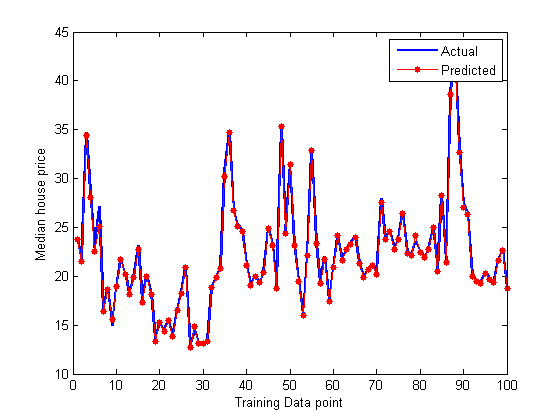Machine Learning with MATLAB

# Regression with Boosted Decision Trees

In this example we will explore a regression problem using the Boston House Prices dataset available from the UCI Machine Learning Repository.

```filename = 'housing.txt';
urlwrite('http://archive.ics.uci.edu/ml/machine-learning-databases/housing/housing.data',filename);
outputNames = {'MEDV'};
housingAttributes = [inputNames,outputNames];
```

## Import Data

Once the file is saved, you can import data into MATLAB as a table using the Import Tool with default options. Alternatively you can use the following code which can be auto generated from the Import Tool:

```formatSpec = '%8f%7f%8f%3f%8f%8f%7f%8f%4f%7f%7f%7f%7f%f%[^\n\r]';
fileID = fopen(filename,'r');
dataArray = textscan(fileID, formatSpec, 'Delimiter', '', 'WhiteSpace', '',  'ReturnOnError', false);
fclose(fileID);
housing = table(dataArray{1:end-1}, 'VariableNames', {'VarName1','VarName2','VarName3','VarName4','VarName5','VarName6','VarName7','VarName8','VarName9',
'VarName10','VarName11','VarName12','VarName13','VarName14'});

% Delete the file and clear temporary variables
clearvars filename formatSpec fileID dataArray ans;
delete housing.txt
```

```housing.Properties.VariableNames = housingAttributes;
X = housing{:,inputNames};
y = housing{:,outputNames};
```## Train a Regression Tree Using the Housing Data

```rng(5); % For reproducibility

% Set aside 90% of the data for training
cv = cvpartition(height(housing),'holdout',0.1);

t = RegressionTree.template('MinLeaf',5);
mdl = fitensemble(X(cv.training,:),y(cv.training,:),'LSBoost',500,t,...
'PredictorNames',inputNames,'ResponseName',outputNames{1},'LearnRate',0.01);

L = loss(mdl,X(cv.test,:),y(cv.test),'mode','ensemble');
fprintf('Mean-square testing error = %f\n',L);
```
```Mean-square testing error = 7.056746
```

## Plot Fit Against Training Data

```figure(1);
% plot([y(cv.training), predict(mdl,X(cv.training,:))],'LineWidth',2);
plot(y(cv.training),'b','LineWidth',2), hold on
plot(predict(mdl,X(cv.training,:)),'r.-','LineWidth',1,'MarkerSize',15)

% Observe first hundred points, pan to view more
xlim([0 100])
legend({'Actual','Predicted'})
xlabel('Training Data point');
ylabel('Median house price');
```## Plot Predictor Importance

Plot the predictors sorted on importance.

```[predictorImportance,sortedIndex] = sort(mdl.predictorImportance);
figure(2);
barh(predictorImportance)
set(gca,'ytickLabel',inputNames(sortedIndex))
xlabel('Predictor Importance')
```## Plot Error

```figure(3);
trainingLoss = resubLoss(mdl,'mode','cumulative');

testLoss = loss(mdl,X(cv.test,:),y(cv.test),'mode','cumulative');
plot(trainingLoss), hold on
plot(testLoss,'r')
legend({'Training Set Loss','Test Set Loss'})
xlabel('Number of trees');
ylabel('Mean Squared Error');
set(gcf,'Position',[249 634 1009 420])
```## Regularize and Shrink the Ensemble

We may not need all 500 trees to get the full accuracy for the model. We can regularize the weights and shrink based on a regularization parameter

```% Try two different regularization parameter values for lasso
mdl = regularize(mdl,'lambda',[0.001 0.1]);
disp('Number of Trees:')
disp(sum(mdl.Regularization.TrainedWeights > 0))
```
```Number of Trees:
194   128
```

Shrink the ensemble using `Lambda = 0.1`

```mdl = shrink(mdl,'weightcolumn',2);

disp('Number of Trees trained after shrinkage')
disp(mdl.NTrained)
```
```Number of Trees trained after shrinkage
128
```

When datasets are large, using a fewer number of trees and fewer predictors based on predictor importance will result in fast computation and accurate results.